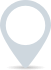# Rate of return calculator

To calculate the rate of return, you’ll need the initial investment value, the final investment value, and the time period over which the investment was held.£
.00
£
.00
- years

This calculator is intended for illustration purposes only and exact payment terms should be agreed with a lender before taking out a loan.

Rate of return

-

## How to calculate rate of return

The formula for calculating the rate of return is as follows:

Rate of Return=(Final Value−Initial Value)/Initial Value×100

Here’s how to calculate it step-by-step:

1. Subtract the Initial Value from the Final Value. This will give you the total change in value over the investment period.
2. Divide the result from step 1 by the Initial Value. This will give you the relative change as a decimal.
3. Multiply the result from step 2 by 100 to convert it into a percentage.

### Clever finance tips and the latest news

#### delivered to your inbox, every week